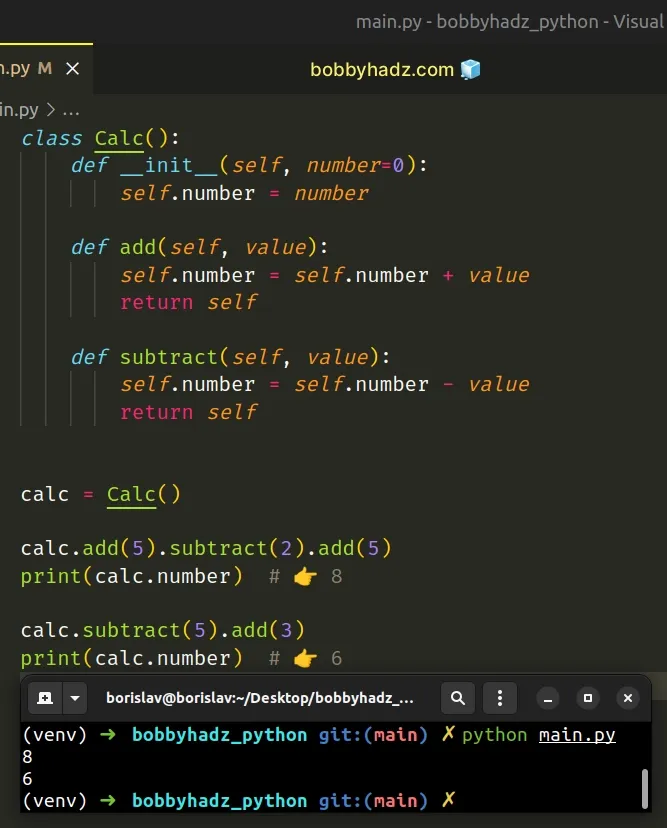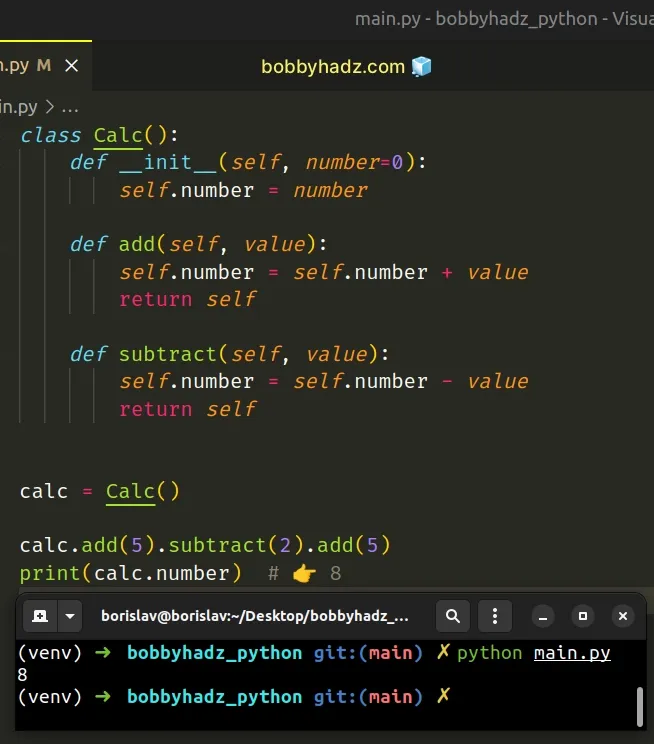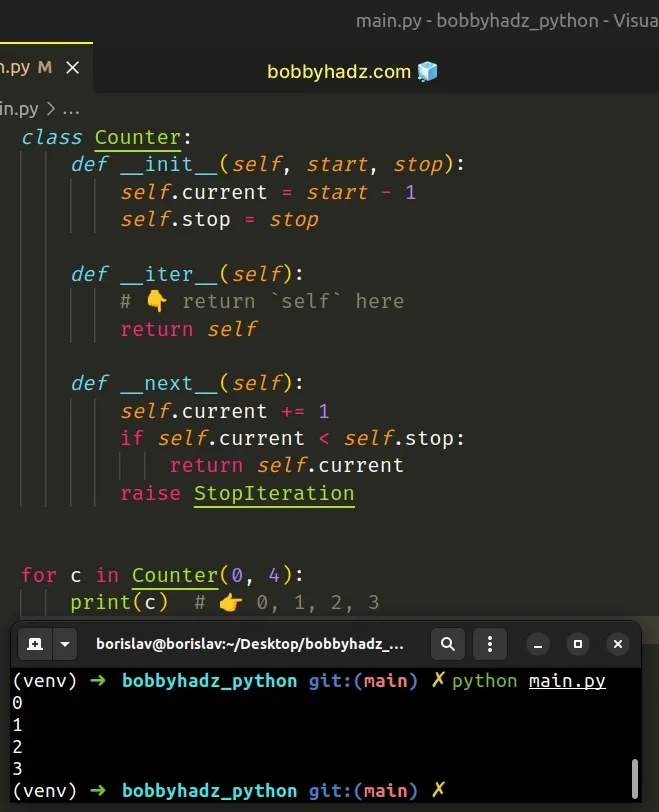# Purpose of 'return self' from a class method in PythonLast updated: Feb 22, 2023
2 min## #Purpose of 'return self' from a class method in Python

The purposes of the `return self` statement from a class method are:

1. The ability to chain multiple calls to a method because `return self` returns the instance object.
2. Using the iterator protocol, which requires us to `return self` from the `__iter__()` method.
main.py
```Copied!```class Calc():
def __init__(self, number=0):
self.number = number

self.number = self.number + value
return self

def subtract(self, value):
self.number = self.number - value
return self

calc = Calc()

print(calc.number)  # 👉️ 8

print(calc.number)  # 👉️ 6
``````The `add` and `subtract` methods in the `Calc()` class use a return self statement.

When we call an instance method, Python automatically passes `self` as the first argument to the method.

`self` represents an instance of the class - the instance on which the method was called.

When we `return self` from a class method, we basically return the instance object.

This allows us to chain multiple calls to the method in a single statement.

## #Chaining multiple calls to a method that returns `self`

The `add()` and `subtract()` methods return the instance, so we can chain many calls to the methods in a single line, without storing the results in intermediary variables.

main.py
```Copied!```class Calc():
def __init__(self, number=0):
self.number = number

self.number = self.number + value
return self

def subtract(self, value):
self.number = self.number - value
return self

calc = Calc()

print(calc.number)  # 👉️ 8
``````You aren't going to see the pattern of method chaining often, but some libraries make use of it.

The idea is that each method returns an object, which allows the calls to be chained together in a single statement without storing the results in intermediary variables.

When you see syntax such as `obj.a().a().b()`, the code under the hood uses the method chaining pattern.

However, returning `self` from class methods is much more common when implementing the iterator protocol.

The __iter__() method must return the `iterator` object itself.

Here is an example of how to make a class iterable by implementing the `__iter__()` method.

main.py
```Copied!```class Counter:
def __init__(self, start, stop):
self.current = start - 1
self.stop = stop

def __iter__(self):
# 👇️ return `self` here
return self

def __next__(self):
self.current += 1
if self.current < self.stop:
return self.current
raise StopIteration

for c in Counter(0, 4):
print(c)  # 👉️ 0, 1, 2, 3
``````The `__iter__()` method is implicitly called at the start of loops and returns the iterator object (`self`).

The `__next__()` method is implicitly called at each loop increment and returns the next value.